# Algebra 1 : How to graph a quadratic function

## Example Questions

### Example Question #1 : How To Graph A Quadratic Function

What is the minimum possible value of the expression below?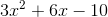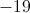The expression has no minimum value.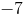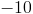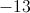Explanation:

We can determine the lowest possible value of the expression by finding the-coordinate of the vertex of the parabola graphed from the equation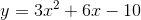. This is done by rewriting the equation in vertex form.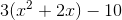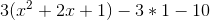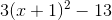The vertex of the parabola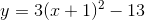is the point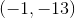.

The parabola is concave upward (its quadratic coefficient is positive), so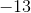represents the minimum value of. This is our answer.

### Example Question #6 : Graphing Parabolas

What is the vertex of the function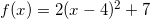? Is it a maximum or minimum?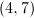; minimum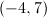; maximum; minimum; maximum; minimum

Explanation:

The equation of a parabola can be written in vertex form: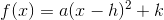.

The point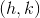in this format is the vertex. Ifis a postive number the vertex is a minimum, and ifis a negative number the vertex is a maximum.In this example,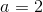. The positive value means the vertex is a minimum.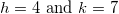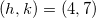### Example Question #4 : Graphing Polynomial Functions

Which of the graphs best represents the following function?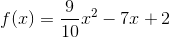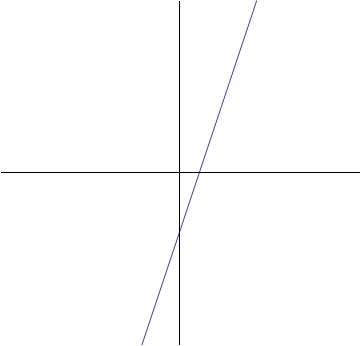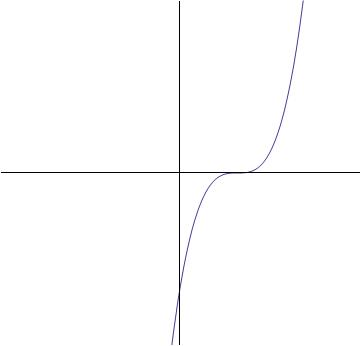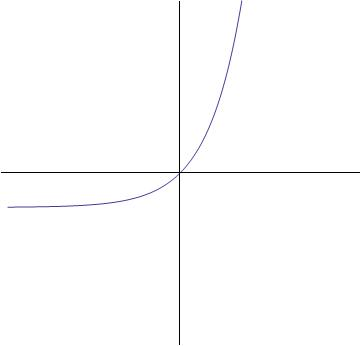None of these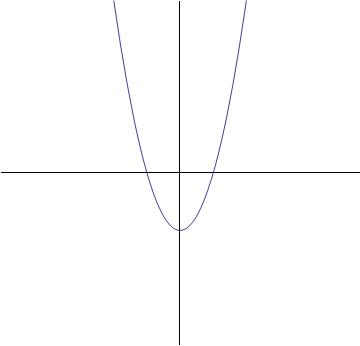Explanation:The highest exponent of the variable term is two (). This tells that this function is quadratic, meaning that it is a parabola.

The graph below will be the answer, as it shows a parabolic curve.### Example Question #2 : How To Graph A Quadratic Function

What is the equation of a parabola with vertex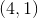and-intercept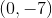?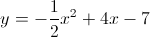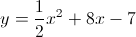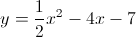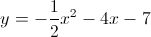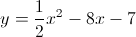Explanation:

From the vertex, we know that the equation of the parabola will take the form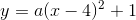for some.

To calculate that, we plug in the values from the other point we are given,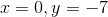, and solve for: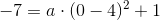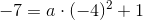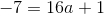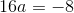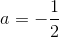Now the equation is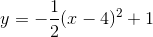. This is not an answer choice, so we need to rewrite it in some way.

Expand the squared term: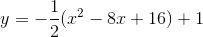Distribute the fraction through the parentheses: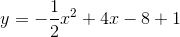Combine like terms: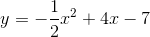### Example Question #8 : Graphing Polynomial Functions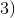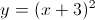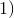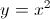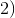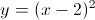None of the above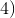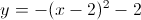Explanation:

Starting with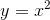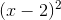moves the parabolabyunits to the right.

Similarly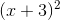moves the parabola byunits to the left.

Hence the correct answer is option.

### Example Question #1 : How To Graph A Quadratic Function

Which of the following graphs matches the function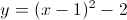?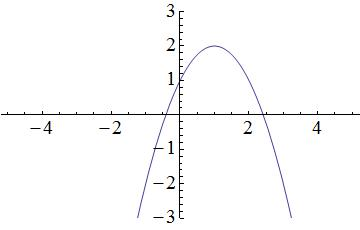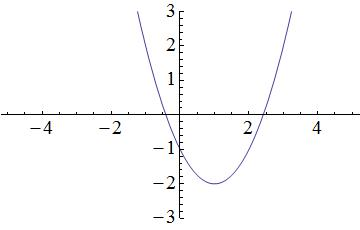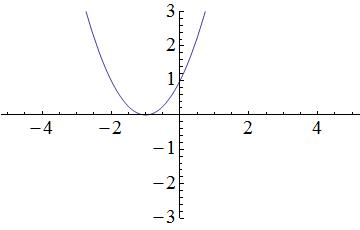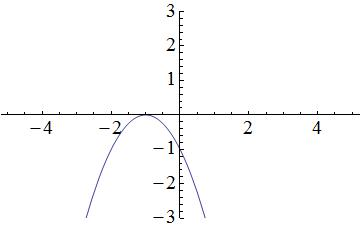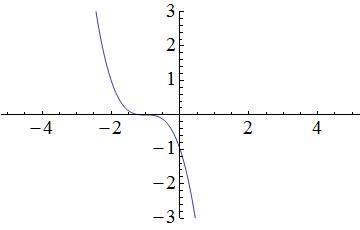Explanation:

Start by visualizing the graph associated with the function: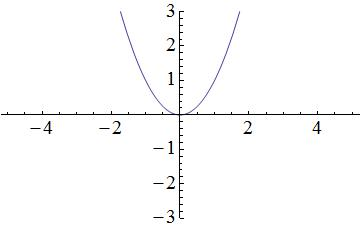Terms within the parentheses associated with the squared x-variable will shift the parabola horizontally, while terms outside of the parentheses will shift the parabola vertically. In the provided equation, 2 is located outside of the parentheses and is subtracted from the terms located within the parentheses; therefore, the parabola in the graph will shift down by 2 units. A simplified graph of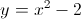looks like this: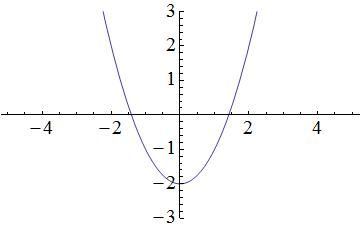Remember that there is also a term within the parentheses. Within the parentheses, 1 is subtracted from the x-variable; thus, the parabola in the graph will shift to the right by 1 unit. As a result, the following graph matches the given function: